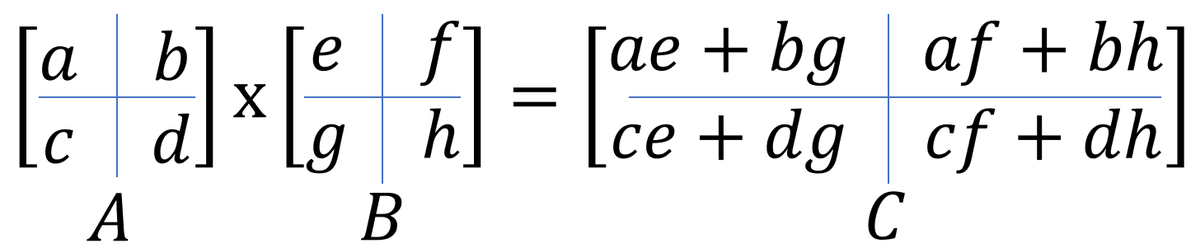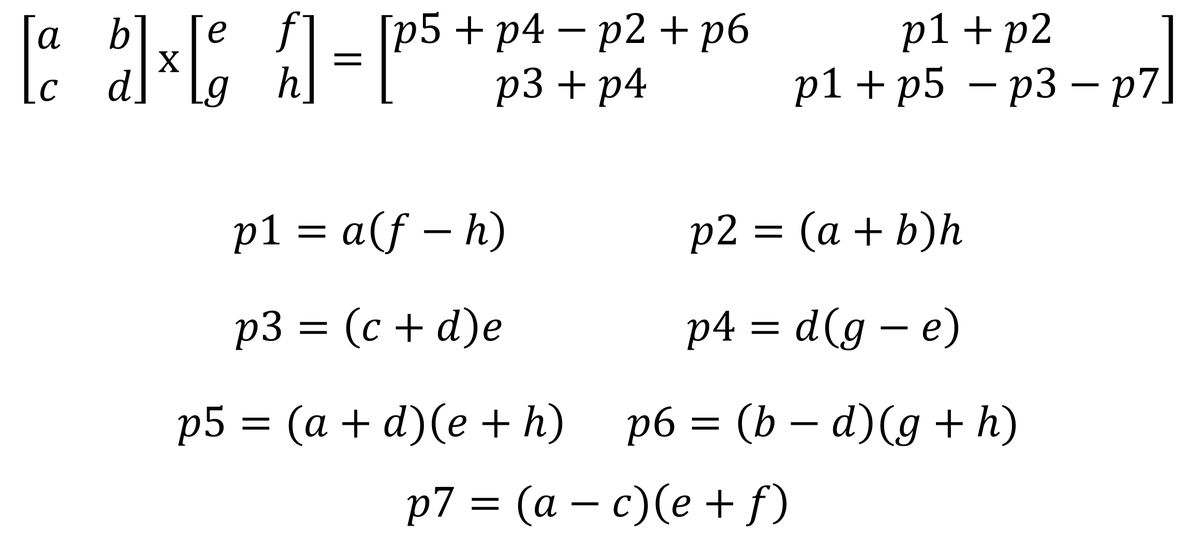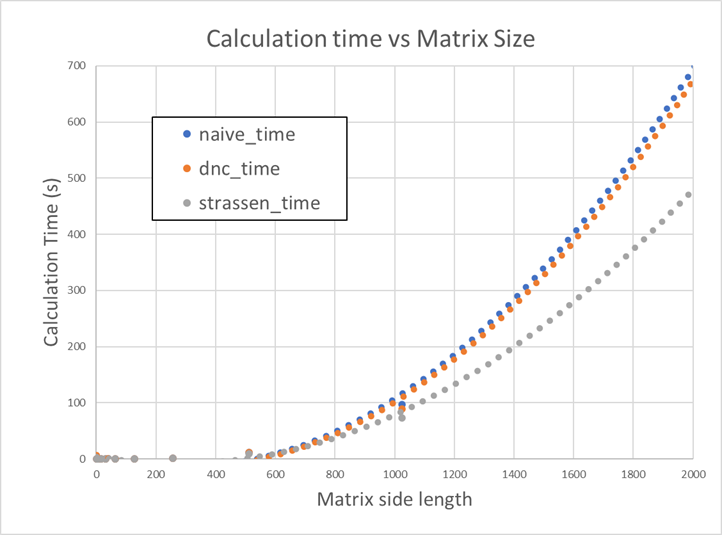# Introduction

Hi! This is Mark Bajo and I am here to share an algorithm for fast multiplication of square matrices. Implementing fast and efficient algorithms is one of the many skills a computer scientist is expected to have. In machine learning and/or deep learning, we deal with large matrices and their computations. In the modern day, we have GPU to compute large matrix operations and even though implementing a naive algorithm on a GPU is still fast, there is still a need for optimizing algorithms implemented on GPU. In this article, we will focus on the core idea of optimizing square matrix multiplication.

# Contents

• Naive Algorithm
• Divide-and-conquer Algorithm
• Strassen Algorithm
• Experiments
• Conclusion

# Naive Algorithm

Below is the naive implementation of matrix multiplication. Because of the three for loops each running a calculation n times, we conclude that the naive solution runs at.

def multiply(A: List[List[float]], B: List[List[float]]):
n = len(A)
C = [[0 for _ in range(n)] for _ in range(n)]
for i in range(n):
for k in range(n):
for j in range(n):
C[i][j] += A[i][k] * B[k][j]
return C


# Divide-and-Conquer Algorithm

Now we take things to the next level. We use a divide-and-conquer approach and try optimizing the naive algorithm. We list down the steps below.

## Matrix Multiplication Divide-and-Conquer Algorithm:

Assuming we are dealing with A x B and each matrix have N by N shape,

• We split each matrix A and B into four submatrices, each submatrix having N/2 by N/2 shape.
• Calculate submatrix multiplication.
• We recurse until the submatrix shape is 1 x 1.Implementing this in pseudo-code,

def multiply_matrix(A: List[List[float]], B: List[List[float]]) -> List[List[float]]:
n = len(A)
C = [[0 for _ in range(n)] for _ in range(n)]
# Base case
if n == 1:
C = A * B
else:
# Split Matrix
a, b, c, d = split_matrix(A)
e, f, g, h = split_matrix(B)

# Colate results for submatrices
# Recurse until 1 x 1
multiply_matrix(a, e), # subproblem
multiply_matrix(b, g)
)

multiply_matrix(a, f),
multiply_matrix(b, h)
)

multiply_matrix(c, e),
multiply_matrix(d, g)
)

multiply_matrix(c, f),
multiply_matrix(d, h)
)

# Combine results
C = combine_results(C_00, C_01, C_10, C_11)
return C


## Time Complexity

Notice that for each part ofsubmatrix, we are conducting multiplication and addition of matrix. In addition, the time complexity is. For the multiplication part, we will have to apply Master Theorem. This results in this equation:which implies that the multiplication part alone has. Therefore, the whole matrix multiplication algorithm hastime complexity which is the same as the naive algorithm's time complexity. Can we do better than this? Yes! It turns out that we can reduce the number of subproblems in this recursion from 8 to 7. This is where Strassen's algorithm comes in.

# Strassen Algorithm

## Implementation

As mentioned in the previous part of this article, the Strassen Algorithm uses 7 subproblems only. The computations for the 7 subproblems are listed below.Implementing this in pseudo-code, we get something like this:

def strassen_multiplication(A: List[List[float]], B: List[List[float]]) -> List[List[float]]:
n = len(A)
C = [[0 for _ in range(n)] for _ in range(n)]
# Base case
if n == 1:
C = A * B
else:
# Split Matrix
a, b, c, d = split_matrix(A)
e, f, g, h = split_matrix(B)

# Colate results for submatrices
p1 = strassen_multiplication(
a,
subtract_matrix(f, h)
)
p2 = strassen_multiplication(
h
)
p3 = strassen_multiplication(
e
)
p4 = strassen_multiplication(
d,
subtract_matrix(g, e)
)
p5 = strassen_multiplication(
)
p6 = strassen_multiplication(
subtract_matrix(b, d),
)
p7 = strassen_multiplication(
subtract_matrix(a, c),
)

# Calculate C submatrices
C_00 = subtract_matrix(
subtract_matrix(p2, p6)
)
C_11 = subtract_matrix(
)

# Combine results
C = combine_results(C_00, C_01, C_10, C_11)
return C


## Time Complexity

Since we have only 7 subproblems, by using Master Theorem, we havewhich translates to time complexity. This is less than!

# Experiments

Important points that I discovered as I was experimenting with the implemented algorithm are the following. * In multiplying small square matrices, I found out that the naive algorithm is the fastest. This might be because of the time it takes to fill in and call the functions in the call stack when we do recursions. We can optimize the divide-and-conquer algorithm by changing the base case. Instead of base case being, we can set it to. Multiplying matrices with sizes the set value, we resort to using the naive algorithm.

def strassen_multiplication(A: List[List[float]], B: List[List[float]], n_switch=128) -> List[List[float]]:
n = len(A)
C = [[0 for _ in range(n)] for _ in range(n)]
# Base case
if n <= n_switch:
C = multiply(A, B)
...

• Below is a graph that shows how long it takes for each algorithm (naive, divide-and-conquer, and Strassen) to finish the inner product calculation.# Conclusion

In this article we have implemented Strassen Algorithm in pure python and experimented on its speed. Thanks to Strassen's Algorithm, we were able to shorten the time it takes to calculate the inner product between two matrices.

• Strassen, Volker (1969). "Gaussian Elimination is not Optimal". Numer. Math. 13 (4): 354–356. doi:10.1007/BF02165411.
• Thomas H. Cormen, Charles E. Leiserson, Ronald L. Rivest, and Clifford Stein. Introduction to Algorithms, Second Edition. MIT Press and McGraw-Hill, 2001. ISBN 0-262-03293-7. Chapter 28: Section 28.2: Strassen's algorithm for matrix multiplication, pp. 735–741.